Question

# Data from student course evaluations were obtained from the Uni- versity of Texas at Austin. The...

Data from student course evaluations were obtained from the Uni- versity of Texas at Austin. The summary statistics are n = 436, x = 3.97, s = 0.55. Use a 0.05 significance level to test the claim that the population of student course evaluations has a mean equal to 4.00. Do the results apply to the population of all students?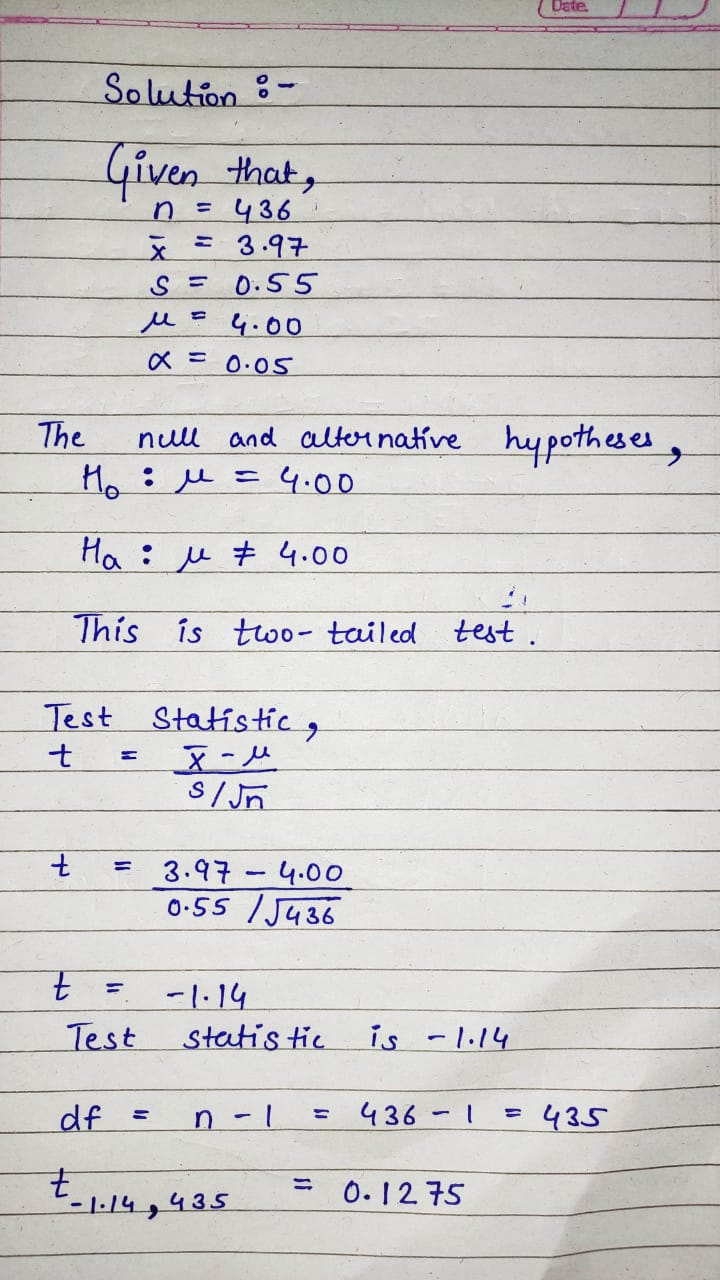#### Earn Coins

Coins can be redeemed for fabulous gifts.

Similar Homework Help Questions
• ### A data set includes data from student evaluations of courses. The summary statistics are n =...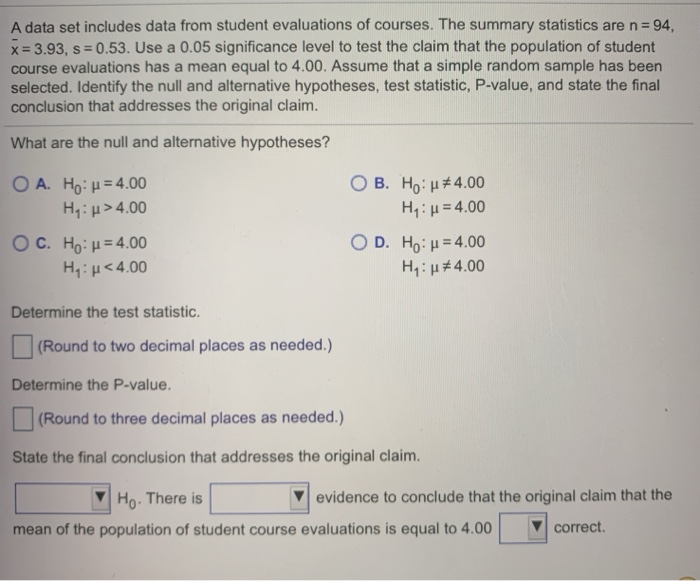A data set includes data from student evaluations of courses. The summary statistics are n = 94. x= 3.93, S = 0.53. Use a 0.05 significance level to test the claim that the population of student course evaluations has a mean equal to 4.00. Assume that a simple random sample has been selected. Identify the null and alternative hypotheses, test statistic, P-value, and state the final conclusion that addresses the original claim. What are the null and alternative hypotheses? O...

• ### Question Help A data set includes data from student evaluations of courses. The summary statistics are...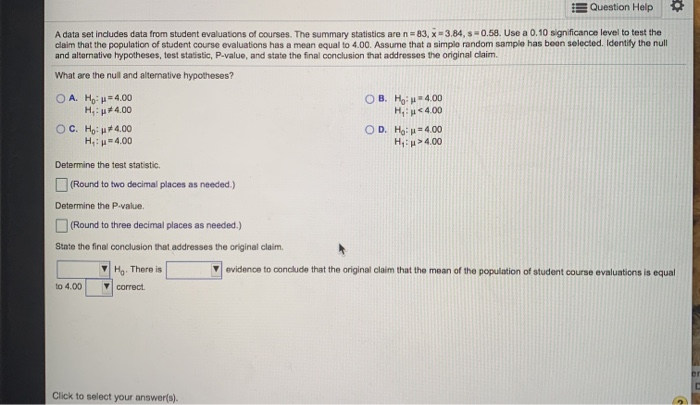Question Help A data set includes data from student evaluations of courses. The summary statistics are n=83, X-3.84, 0.58. Use a 0.10 significance level to test the claim that the population of student course evaluations has a mean equal to 4.00. Assume that a simple random sample has been selected. Identify the null and alternative hypotheses, test statistic, P-value, and state the final conclusion that addresses the original claim. What are the null and alternative hypotheses? OA. Hop=4.00 OB. Ho...

• ### A data set includes data from student evaluations of courses. The summary statistics are nequals=83, x...

A data set includes data from student evaluations of courses. The summary statistics are nequals=83, x overbarxequals=3.95​, sequals=0.68. Use a 0.10 significance level to test the claim that the population of student course evaluations has a mean equal to 4.00. Assume that a simple random sample has been selected. Identify the null and alternative​ hypotheses, test​ statistic, P-value, and state the final conclusion that addresses the original claim.

• ### A data set includes data from student evaluations of courses. The summary statistics are n=87, x̅= 3.43, s=0.65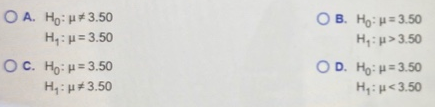A data set includes data from student evaluations of courses. The summary statistics are n=87, x̅= 3.43, s=0.65. Use a 0.05 significance level to test the claim that the population of student course evaluations has a mean equal to 3.50. Assume that a simple random sample has been selected. Identify the null and alternative hypotheses, test statistic, P-value, and state the final conclusion that addresses the original claim. What are the null and alternative hypotheses?

• ### A data set includes data from student evaluations of courses. The summary statistics are n=88, x=...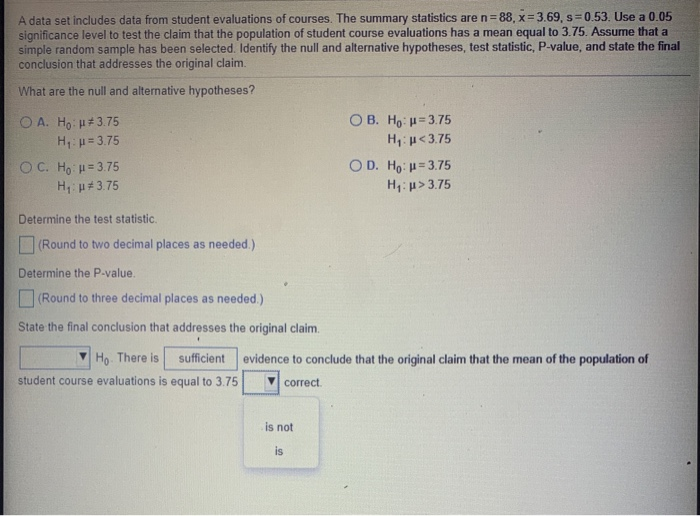A data set includes data from student evaluations of courses. The summary statistics are n=88, x= 3.69, s=0.53. Use a 0.05 significance level to test the claim that the population of student course evaluations has a mean equal to 3.75. Assume that a simple random sample has been selected. Identify the null and alternative hypotheses, test statistic, P-value, and state the final conclusion that addresses the original claim. What are the null and alternative hypotheses? O A. Ho: +3.75 H...

• ### A data set includes data from student evaluations of courses. The summary statistics are nequals=8484​, x...

A data set includes data from student evaluations of courses. The summary statistics are nequals=8484​, x overbarxequals=3.453.45​, sequals=0.640.64 .Use a 0.010.01 significance level to test the claim that the population of student course evaluations has a mean equal to 3.503.50. Assume that a simple random sample has been selected. Identify the null and alternative​ hypotheses, test​ statistic, P-value, and state the final conclusion that addresses the original claim. Determine the test statistic. nothing (Round to two decimal places as​ needed.)

• ### A data set includes data from student evaluations of courses. The summary statistics are n=88, X=3:44,...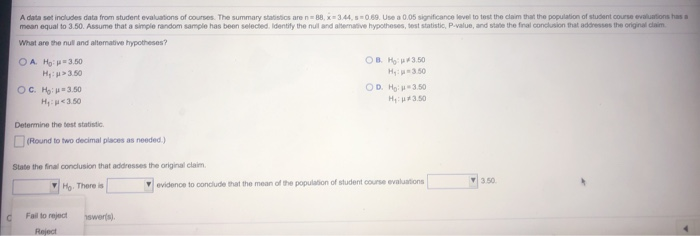A data set includes data from student evaluations of courses. The summary statistics are n=88, X=3:44, 5-0.69 Use a 0.05 significance level to test the claim that the population of student course evaluations has a mean equal to 3.50. Assume that a simple random sample has been selected. Identify the nul and alternative hypotheses, test statistic, P.value, and state the final conclusion that addresses the original claim. What are the null and alterative hypotheses? O A. Ho 3.60 OB H:73.50...

• ### A data set includes data from student evaluations of courses. The summary ticaren 38,361,30.58 Use a...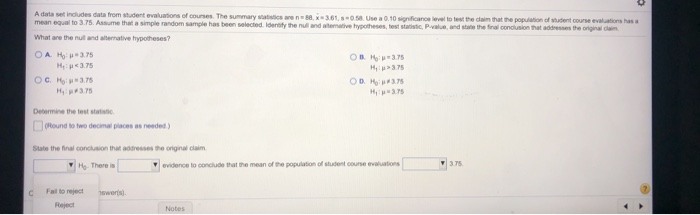A data set includes data from student evaluations of courses. The summary ticaren 38,361,30.58 Use a 0.10 significance level to test the daim that the population of student course evaluation has a mean equal to 3.75. Assume that a simple random sample has been elected. Identify the null and teave hypotheses, tests and state the final conclusion that addresses the original What are the nut and seative hypotheses? OAH 3.75 OB Mo: 3.75 HR3.75 H>3.75 OCH 3.75 OD #375 H...

• ### A data set includes data from student evaluations of courses. The summary statistics are nequals=9393​, x...

A data set includes data from student evaluations of courses. The summary statistics are nequals=9393​, x overbarxequals=3.453.45​, sequals=0.630.63. Use a 0.010.01 significance level to test the claim that the population of student course evaluations has a mean equal to 3.503.50. Assume that a simple random sample has been selected. Identify the null and alternative​ hypotheses, test​ statistic, P-value, and state the final conclusion that addresses the original claim. What are the null and alternative​ hypotheses? A. Upper H 0H0​: muμnot...

• ### 5. A data set includes data from 400 random tornadoes. The display from technology available below...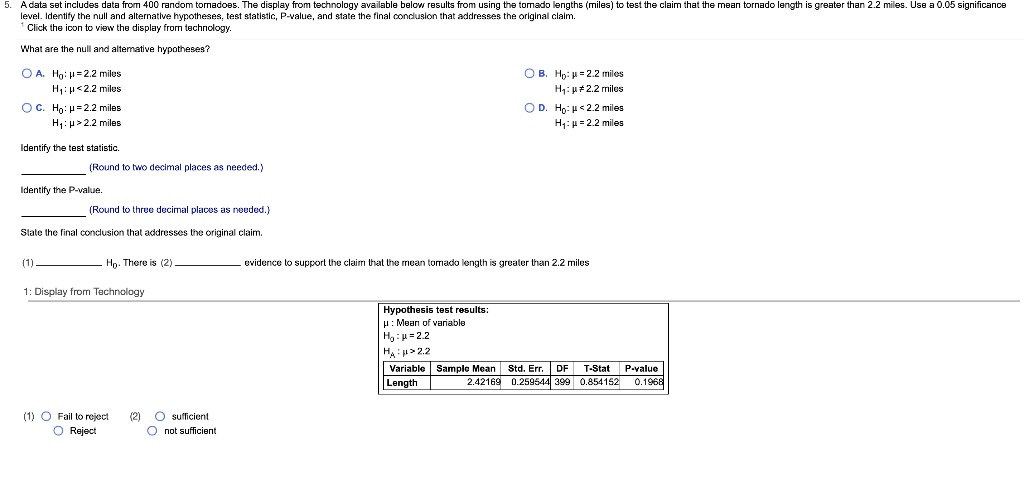5. A data set includes data from 400 random tornadoes. The display from technology available below results from using the tomado lengths (miles) to test the claim that the mean tornado length is greater than 2.2 miles. Use a 0.05 significance level. Identify the null and alternative hypotheses, test statistic, P-value, and state the final conclusion that addresses the original claim. Click the icon to view the display from technology. What are the null and alterative hypotheses? O A. Hoh=2.2...